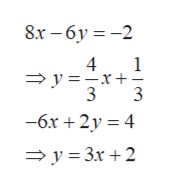# Find an equation for the line perpendicular to the line 8x−6y=-2 having the same y-intercept as −6x+2y=4

Question
45 views

Find an equation for the line perpendicular to the line 8x−6y=-2 having the same y-intercept as −6x+2y=4

check_circle

Step 1

Find the equation of line perpendicular to line 1:

and y-intercept is same as line 2.

Step 2

We know that, slope intercept form of line is :

where m is slope

b is y intercept

Step 3

Write the both lines in slope int...help_outlineImage Transcriptionclose8х - бу %3D-2 4 1 y x+- 3 3 - бх + 2у %3D 4 y 3r 2 fullscreen

### Want to see the full answer?

See Solution

#### Want to see this answer and more?

Solutions are written by subject experts who are available 24/7. Questions are typically answered within 1 hour.*

See Solution
*Response times may vary by subject and question.
Tagged in
MathAlgebra

### Equations and In-equations